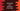# How to join elements of an array in JavaScriptJoining array elements is quite common JavaScript operation. Joining means each element of the array is joined with its previous and next element. In this post, I will show you how to join array elements in JavaScript.

For example, we have the below string array :

[‘one’, ‘two’, ‘three’, ‘four’]

Our program will join these words and print the below string :

one,two,three,four

or,

one-two-three-four

i.e. it will join the array elements using a custom separator.

## Method 1: Using forEach :

We can create one string variable, iterate through the array and join each element to this string variable to create the final string.

Let’s write the code :

``````let givenArray = ['one','two','three']
let result = ''

givenArray.forEach(item => result = result + item + ',')
result = result.slice(0, -1)

console.log(result)``````

Explanation of this program :

1. givenArray is the given array of strings.
2. result is the final result. It is a string initialised with empty value.
3. We are iterating through the array elements one by one using forEach. For each element, we are adding it with result with a comma at end.
4. The final result will add one comma at the end of the string. We are using slice to remove the last comma.

It will print the below output :

``one,two,three``

## Method 2: Using Array.join :

Array.join() method is used to join array elements with a custom separator. I would recommend you to use this method than the above one because it is concise and efficient. The above example uses one loop and slice to get the result but using join, you can get the result in just one line :

``````let givenArray = ['one','two','three']
let result = givenArray.join(',')

console.log(result)``````

That’s it. It will print :

``one,two,three``

Even, if you don’t specify the separator to join, it will add comma as the default separator :

``````let givenArray = ['one','two','three']
let result = givenArray.join()

console.log(result)``````

It will print the same result.